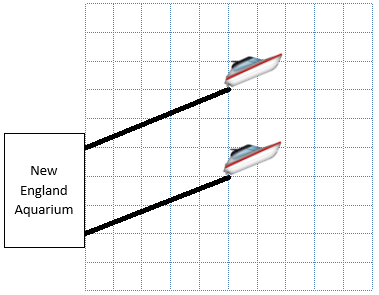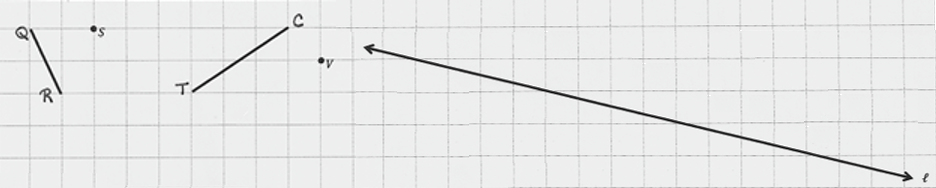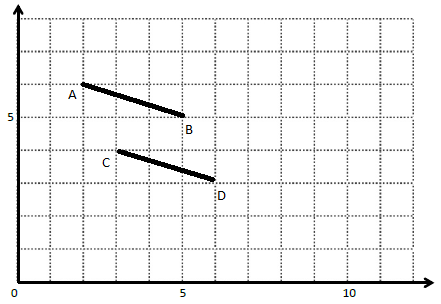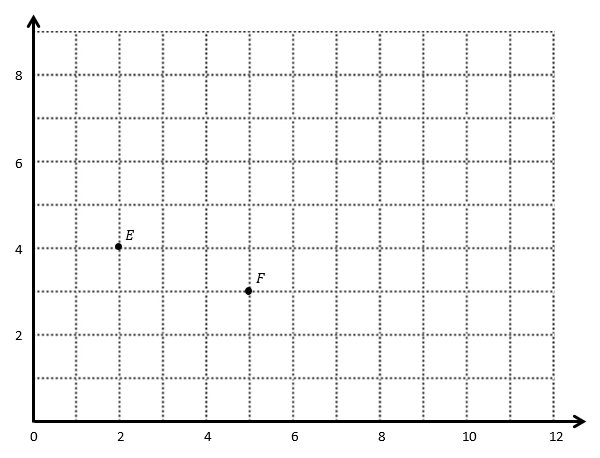# Patterns and the Coordinate Plane

## Objective

Draw parallel lines on a coordinate plane.

## Common Core Standards

### Core Standards

?

• 5.G.A.1 — Use a pair of perpendicular number lines, called axes, to define a coordinate system, with the intersection of the lines (the origin) arranged to coincide with the 0 on each line and a given point in the plane located by using an ordered pair of numbers, called its coordinates. Understand that the first number indicates how far to travel from the origin in the direction of one axis, and the second number indicates how far to travel in the direction of the second axis, with the convention that the names of the two axes and the coordinates correspond (e.g., x-axis and x-coordinate, y-axis and y-coordinate).

• 5.G.A.2 — Represent real world and mathematical problems by graphing points in the first quadrant of the coordinate plane, and interpret coordinate values of points in the context of the situation.

?

• 4.G.A.1

## Criteria for Success

?

1. Determine whether two line segments drawn on a grid or coordinate plane are parallel.
2. Draw parallel line segments on a grid or coordinate plane.
3. Identify the coordinates of points on two parallel line segments.
4. (Optional) Notice patterns in the coordinates of two parallel line segments.

## Tips for Teachers

?

Students have done similar work in Grade 4 Unit 4 Lesson 4 and Lesson 17. This may still be a challenging lesson, though, so it may be useful to refer to Grade 4 Unit 4 to see the ways in which students worked on similar tasks to inform your instruction of this lesson.

#### Fishtank Plus

• Problem Set
• Student Handout Editor
• Vocabulary Package

?

### Problem 1

The New England Aquarium rescues sea animals that are in danger, like sea turtles. On a recent rescue mission, two boats left different docks at the New England Aquarium and headed toward Harbor Island. They want to take parallel paths to the island so that they don’t risk running into each other.Are the two boats’ paths above parallel? How do you know?

### Problem 2

On the coordinate grid below,

1. Draw a line through point S that is parallel to ${\overline{QR}}$.
2. Draw a line through point V that is parallel to ${\overline{TC}}$.
3. Draw a line that is parallel to line l.#### References

EngageNY Mathematics Grade 5 Mathematics > Module 6 > Topic C > Lesson 13Concept Development

Grade 5 Mathematics > Module 6 > Topic C > Lesson 13 of the New York State Common Core Mathematics Curriculum from EngageNY and Great Minds. © 2015 Great Minds. Licensed by EngageNY of the New York State Education Department under the CC BY-NC-SA 3.0 US license. Accessed Dec. 2, 2016, 5:15 p.m..

Modified by Fishtank Learning, Inc.

### Problem 3

Use the coordinate plane below to answer the questions.1. What are the coordinates of points A and B?
2. What are the coordinates of points C and D?
3. Are the two line segments parallel? How do you know?
4. Draw another line segment, ${\overline{EF}}$, on the coordinate plane that is also parallel and the same length.
5. What are the coordinates of point E and point F?
6. Explain how you figured out where to plot ${\overline{EF}}$.

#### References

EngageNY Mathematics Grade 5 Mathematics > Module 6 > Topic C > Lesson 14Concept Development

Grade 5 Mathematics > Module 6 > Topic C > Lesson 14 of the New York State Common Core Mathematics Curriculum from EngageNY and Great Minds. © 2015 Great Minds. Licensed by EngageNY of the New York State Education Department under the CC BY-NC-SA 3.0 US license. Accessed Dec. 2, 2016, 5:15 p.m..

Modified by Fishtank Learning, Inc.

## Problem Set & Homework

### Discussion of Problem Set

• How did you know which line segments were parallel and which were not parallel in #2?
• Is there only one correct answer for each part in #2? If so, why? If not, what are some other possible segments?
• Explain your thought process as you identified the location of point H in #4(f).

?

Use the coordinate plane below for #1 and #2.a.   Draw a line through points E and F.

b.   Draw a second line that is parallel to $\overleftrightarrow{EF}$ but does not contain either point.

#### References

EngageNY Mathematics Grade 5 Mathematics > Module 6 > Topic C > Lesson 14Exit Ticket

Grade 5 Mathematics > Module 6 > Topic C > Lesson 14 of the New York State Common Core Mathematics Curriculum from EngageNY and Great Minds. © 2015 Great Minds. Licensed by EngageNY of the New York State Education Department under the CC BY-NC-SA 3.0 US license. Accessed Dec. 2, 2016, 5:15 p.m..

Modified by Fishtank Learning, Inc.